# Few Mode Fiber Design

The capacity of single-mode fiber optical transmission systems is limited. Spatial Division Multiplexing (SDM) is an attractive option for expanding the channel capacity, in addition to Wavelength Division Multiplexing (WDM) and Polarization Division Multiplexing (PDM). Few-mode fibers (FMFs) as one of the SDM approaches have been investigated in past few years due to their large information transport capacity [1,2].

Synopsys RSoft Photonic Device tools include mode solvers. BeamPROP is a BPM-based mode solver and FemSIM is a FEM-based mode-solver, combined with RSoft’s powerful scanning and post processing capabilities, these are excellent tools to study the critical parameters of FMFs, such as effective index, normalized frequency, normalized propagation constant, mode profile, mode area, dispersion parameters, bending loss and so on. In addition, the challenging task of designing FMFs with low differential mode group delays (DMGDs) can be accomplished with small post-processing tasks. This includes designing FMFs so that all modes can be simultaneously used in multiple input, multiple output (MIMO) technologies and processed with as low s complexity as possible.

This tip demonstrates an example to calculate the critical parameters mentioned above for FMF. Figure 1 shows a trench-assist graded LP6 FMF. This structure receives a good deal of interest because it can reduce the bending sensitivity which become a source of difficulty when the number of LP modes increases. The user profile defined in Equation 1 was used to create the graded trench-assisted fiber. The design strategy is to choose the highest possible normalized frequency value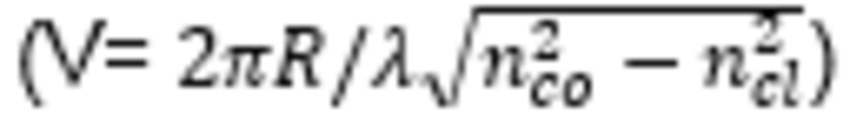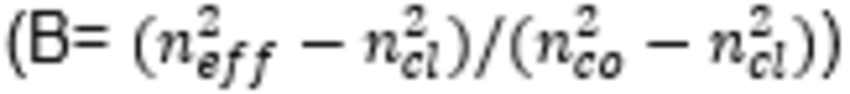to the cut-off of the next higher-order LP modes so that LP modes will have a normalized propagation constant closest to their highest possible values, in order to have less bending loss.

Once V is set, then one can optimize the core radius R, shape factor α, mode area to have low DMGDs, low bending loss, and small intra-mode nonlinearity.

In this tip, we will demonstrate how to calculate the parameters mentioned above with RSoft’s mode solver. Note that all design files can be accessed from the Customer Support Portal.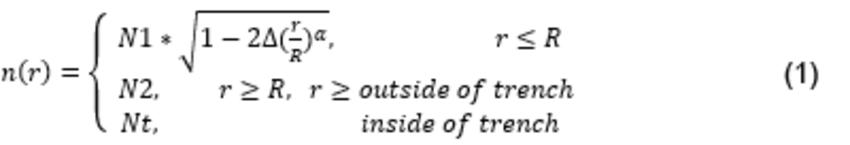Where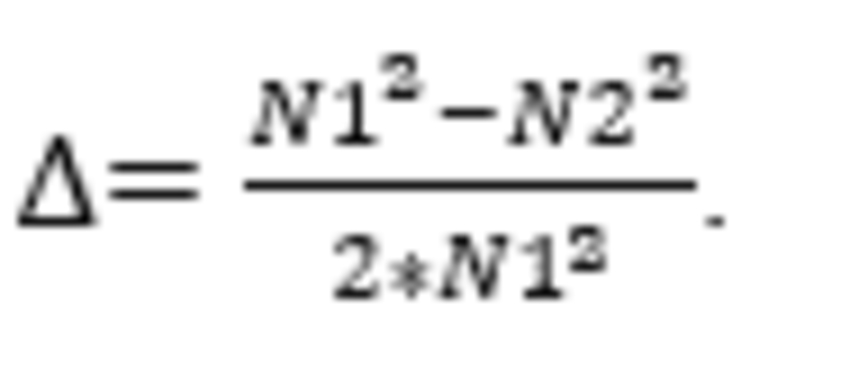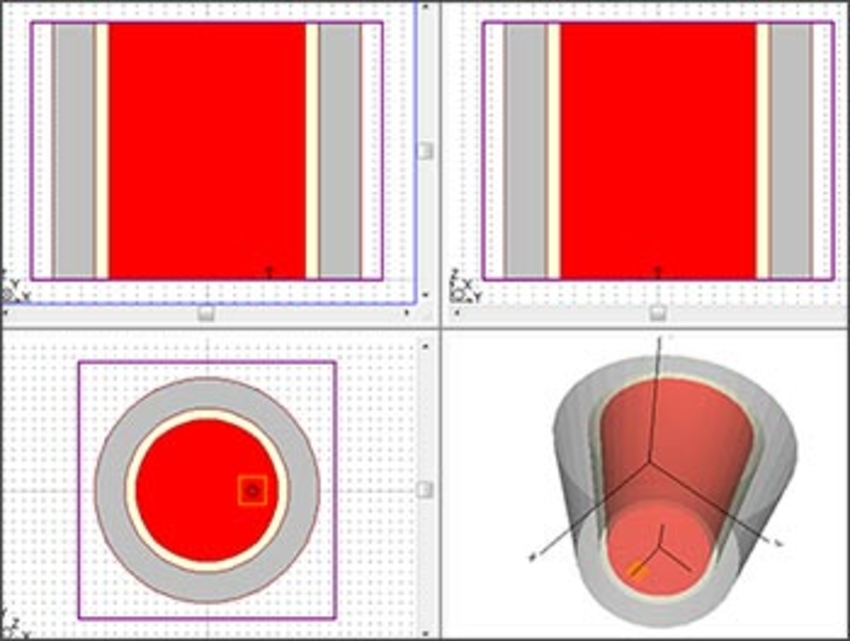(a)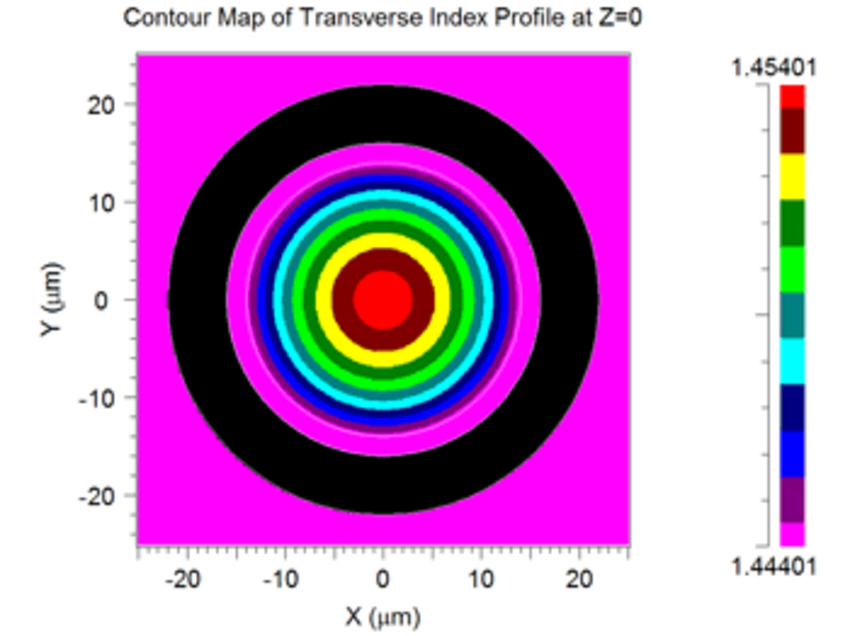(b)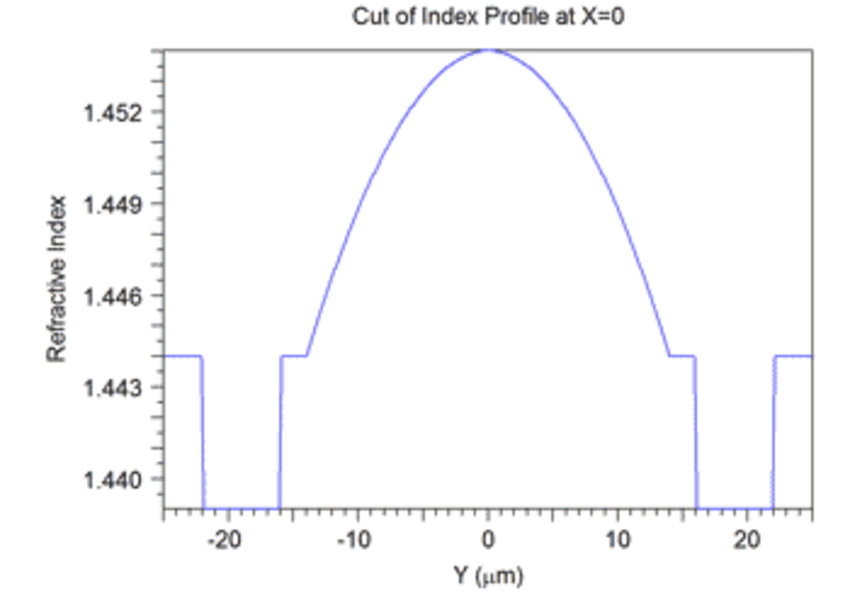(c)

Figure 1: (a) Structure setup in RSoft CAD Environment, (b) Cross-sectional index profile, (c) Index plot of a cut plane

## 1. Mode Profiles

The BPM correlation-method mode solver was used to calculate the LP modes of this low-contrast FMF. An offset launch field and a long propagation distance were used to ensure all modes were found. For α=1.95, we obtained the 6 LP modes.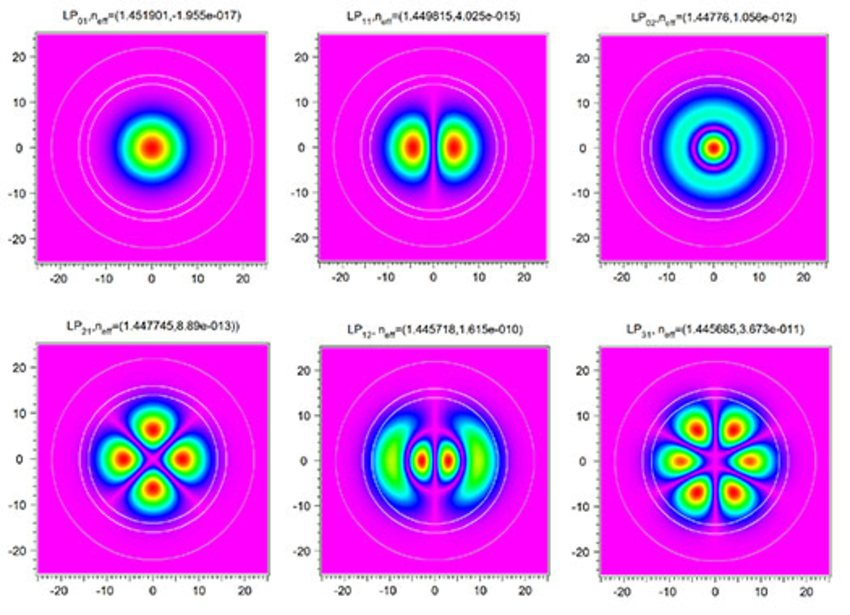Figure 2: The 6 LP mode profiles

## 2. BV Curve

While we calculate the LP modes, we created MOST metric functions to simultaneously obtain B and V, and then extract the B and V values to make a B-V plot.  Figure 3a shows a B-V curve when the wavelength is scanned from 1 to 2 um in MOST, calculating the modes at each step.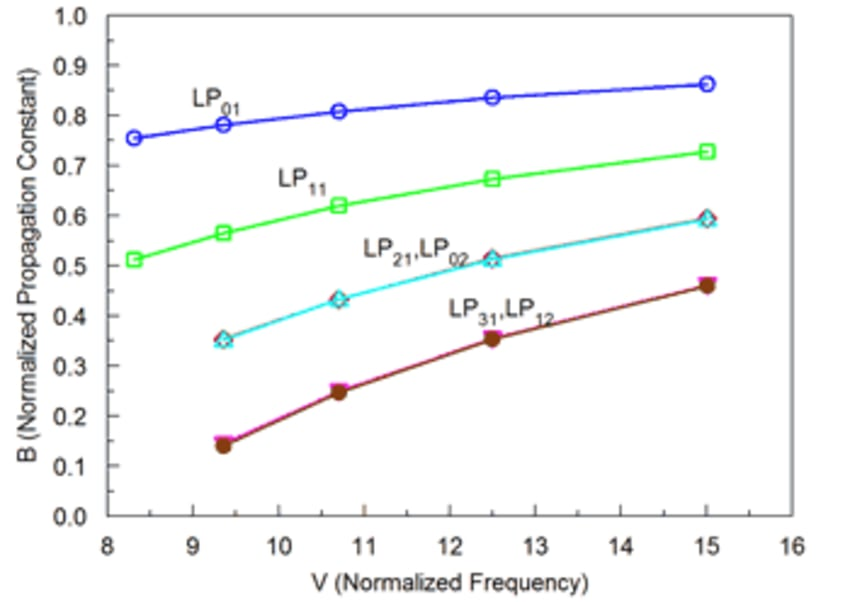(a)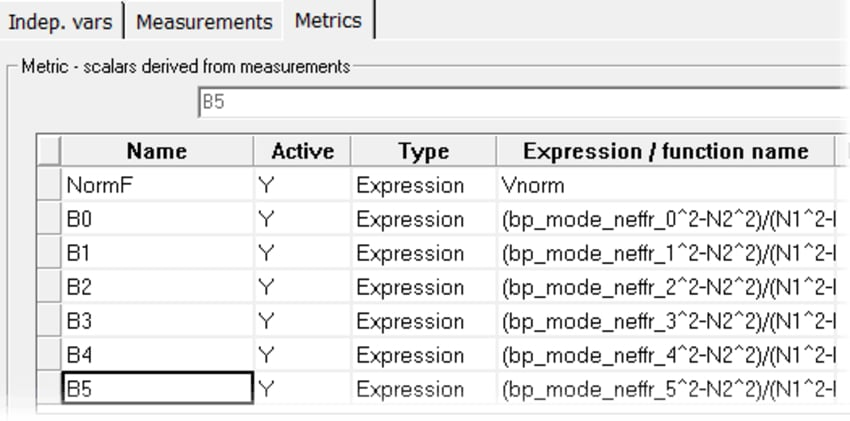(b)

Figure 3: (a) The B-V curve obtained by scanning wavelength in MOST.
(b) Metrics setups in MOST

## 3. Bending Losses

The bending loss directly defines how many modes can be used for an FMF. It is normally evaluated by the loss dB for one turn: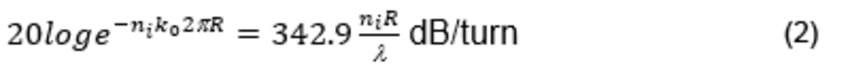This parameter can again be obtained via MOST metrics, using the imaginary part of the effective indices. Figure 4 shows the losses per turn for these 6 LP modes.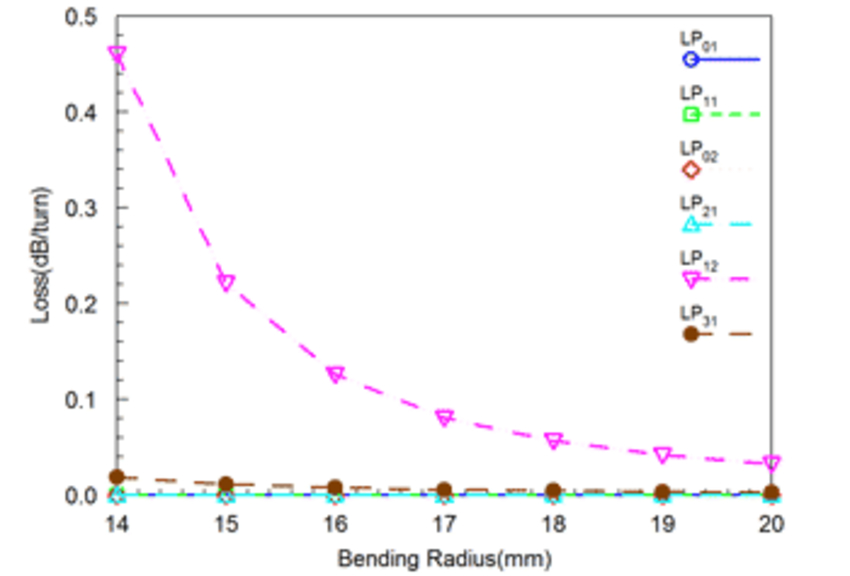(a)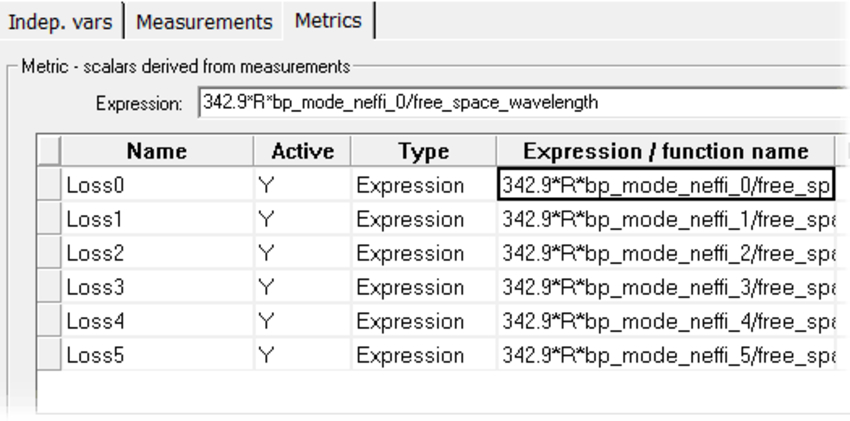(b)

Figure 4: (a) The losses of each mode per turn vs. the bending radius.
(b) Metrics setups in MOST.

## 4. Mode Area

Mode area is also an important parameter in FMF. A small mode area may increase nonlinear sensitivity, while too large a mode area may increase bending loss. The mode area, defined in the equation below, can be calculated using the overlap integral option of RSoft’s bdutil utility combined with mode calculation. We created a batch file to automate this operation. Figure 5 shows the mode area changes vs. wavelength for these 6 LP modes.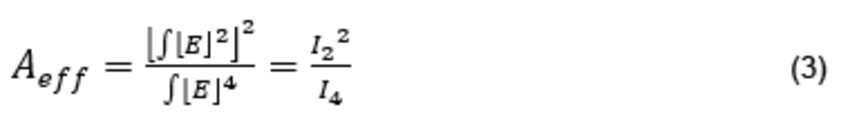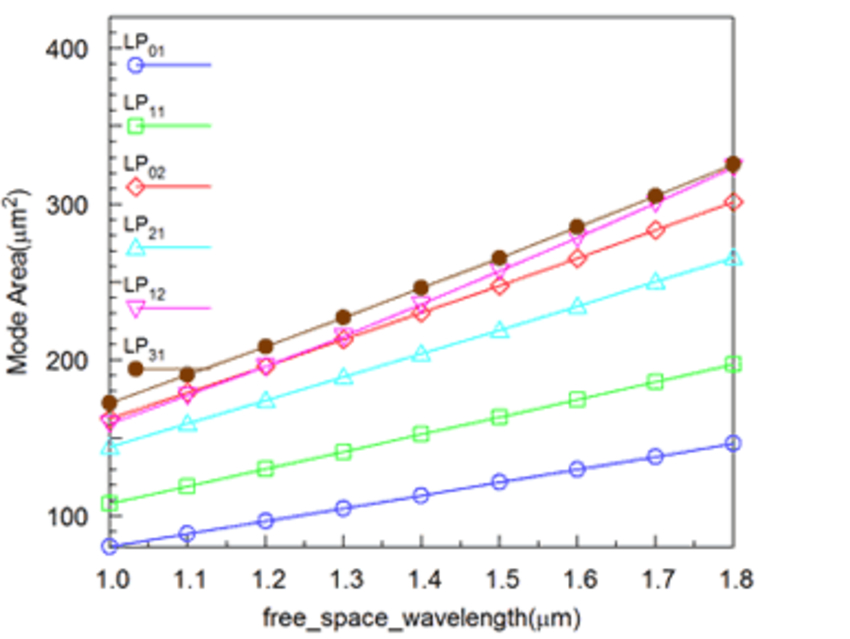(a)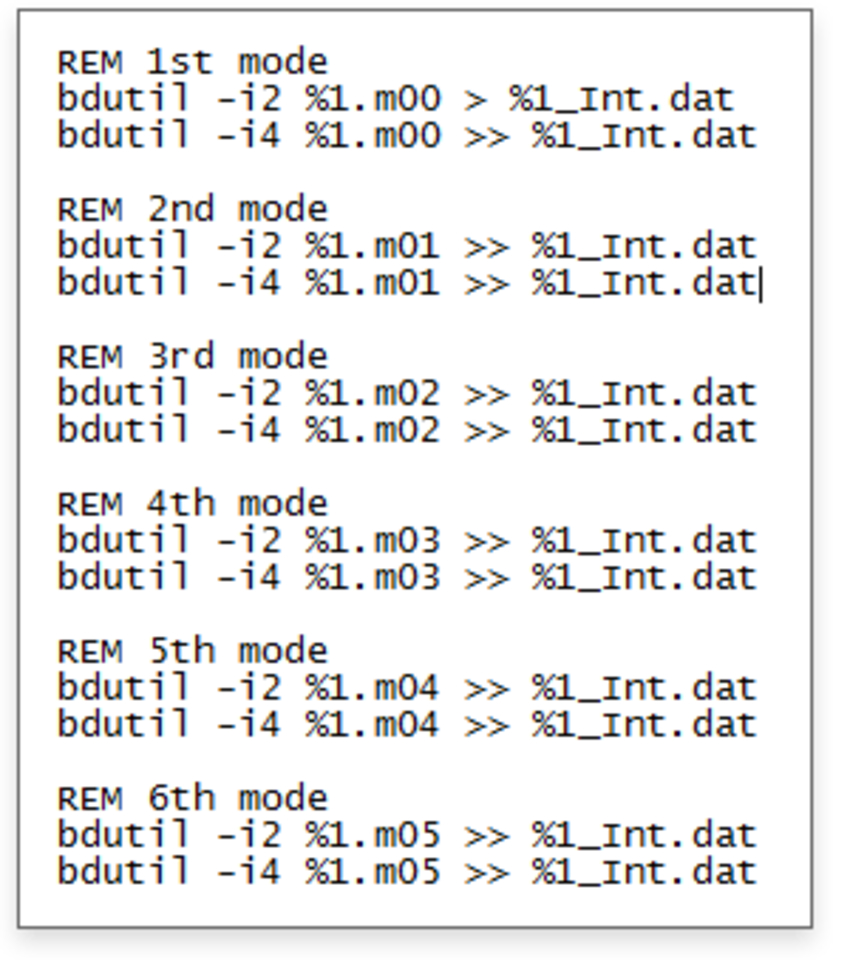(b)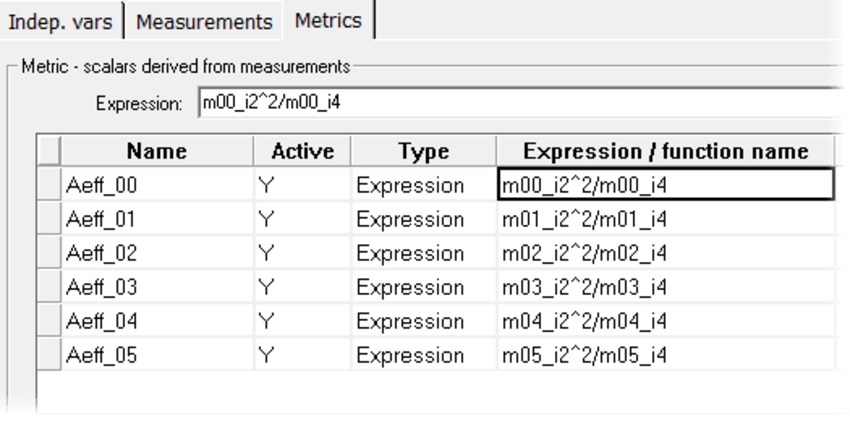(c)

Figure 5: (a) Effective mode areas changes vs. wavelength; (b) The batch program input in MOST post process; (c) Metrics setups in MOST.

## 5. Dispersion of LP Modes

Waveguide dispersion directly impacts the performance of the communication system. RSoft’s disperse utility directly calculates the group index and dispersion parameters after a mode calculation vs. wavelength scan. Based on the group index of each mode, we created a simple script to calculate the differential mode group delay (DMGD), which is conventionally defined as group index difference of higher waveguide modes vs. the group index of fundamental mode. Low DMGD FMFs adapt to strongly coupled mode division multiplexed systems.

Figures 6a and 6b show the effective index, group index and DMGD of this LP6 FMF vs. wavelength, respectively. DMGD is calculated with the script shown in Figure 6c. The table shows some of the main design parameters for this LP6 FMF, at 1.55 µm wavelength.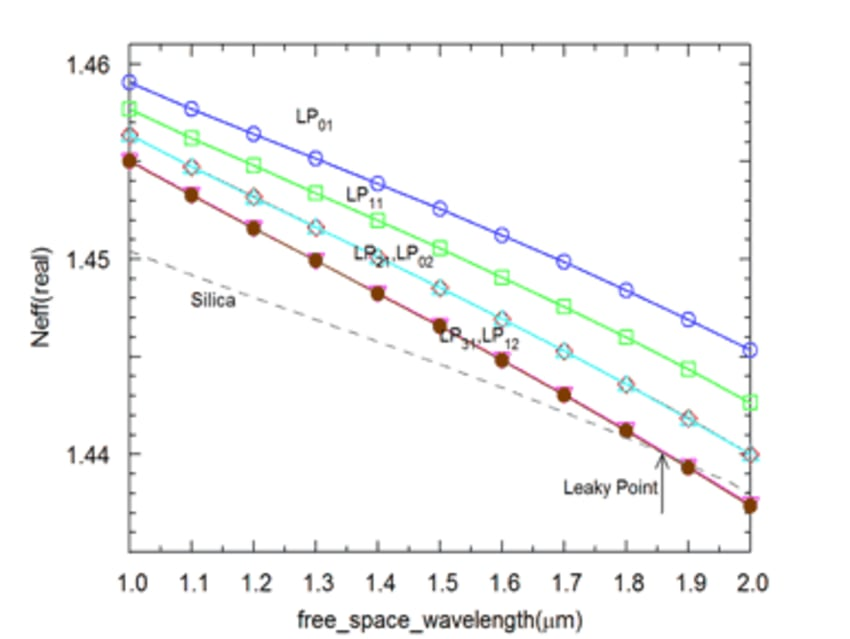(a)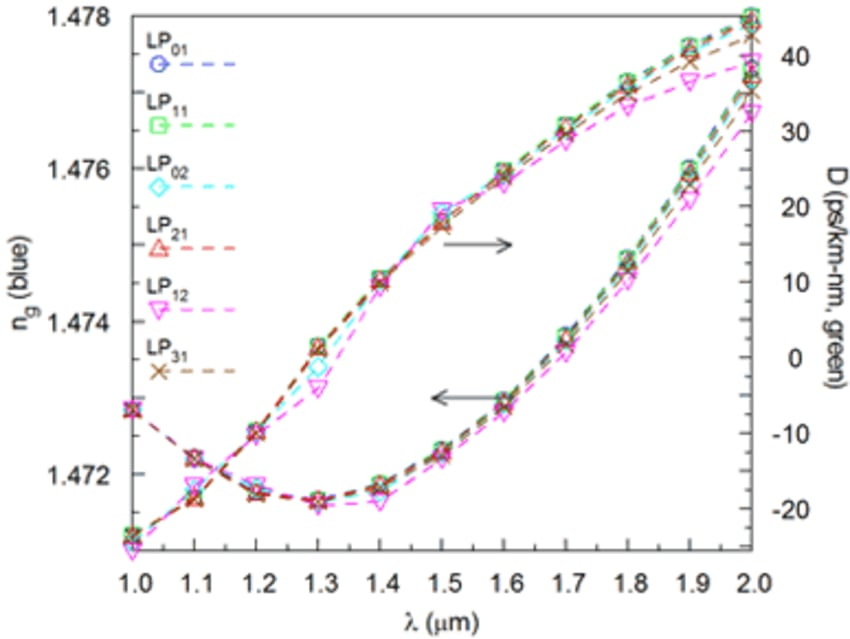(b)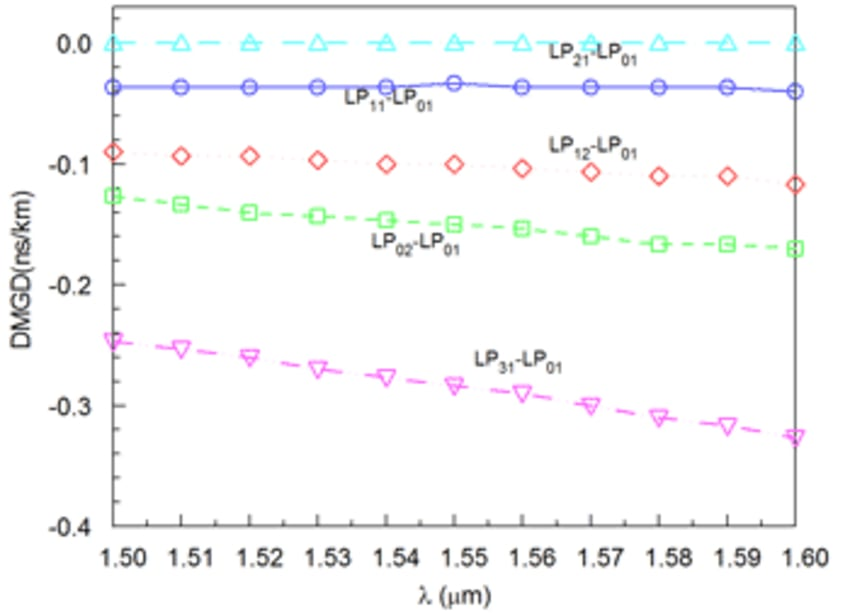(c)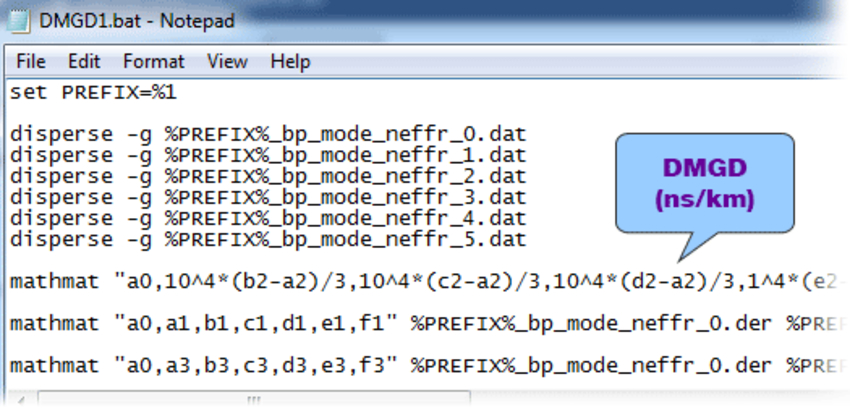(d)

 LP01 LP11 LP21s LP02 LP31s LP12 Neff - Ncl (x10-3s) 7.897 5.805 3.733 3.75 1.675 1.708 Bend Loss dB/1cm radius turn <<1 <<1 <<1 <<1 <1 <1 DMGD ps/km -- -0.03 -0.14 -0.09 -0.26 -0.48 MAX|DMGD| ps/km 0.45 ( LP11s   vs.   LP12 ) Aeff (um2) 125.68 169.69 227.07 255.33 272.23 277.24 Dispersion ps/nm/km 21.7 21.62 21.6 21.3 20.9 21.0

(e)

Figure 6: Dispersion properties of LP6 fiber. (a) Effective index changes vs wavelength; (b) Group index and Dispersion parameters change vs. wavelength; (c) DMGD between higher modes and fundamental mode vs. wavelength; (d) Batch program for DMGD calculation; (e) Optical specs of LP6 fiber at 1.55 um.

Download simulation files (zipped file, 14 KB) from the Customer Portal (account required)